# namniart

## Feb 16, 2014 - Path Planning for Dagny

I’ve spent the last few months experimenting with path planning for Dagny.

## Background

Dagny is an car-type robot, which means that I have a very constrained set of possible motions. In particular, I can move forwards and backwards, and can turn while moving, but can’t turn in place or move sideways. If you’ve ever driven a car and tried to parallel park, you can understand how difficult it can be to maneuver a car-type vehicle.

These motion constraints mean that I have to make sure that the motion planners understand the motion constraints and can generate a path that doesn’t have turn-in-place behaviors, and honors the minimum turning radius of the robot.

The overall plan to achieve this is to use the ROS navigation stack with the sbpl_lattice_planner as the global planner with custom motion primitives and a custom local planner.

## SBPL

SBPL is the Search-Based Planning Lab, and they publish a set of planning libraries called lattice planners that discretize the state space, and define motions that connect one point and angle on the grid to another grid point. These motions are usually either a straight line, or a combination of a straight line and an arc, to achieve both the desired linear and angular offset between poses. Once the motion primitives are defined, they form a graph that can be searched with an algorithm such as A* to produce the desired path.

Since the motion primitives define the search space and how the robot moves, having a good set of motion primitives is critical to producing good plans.

## Generating Motion Primitives

My strategy for generating motion primitives revolves around building primitives from combinations of three types of actions:

• Linear segments with zero angular velocity.
• Arc segments with constant angular velocity
• Spiral segments with varying angular velocity and fixed angular acceleration.

Since Dagny’s dynamics allow me to execute any turn at any speed, I can ignore linear velocity and acceleration in my models. Therefore, for the sake of simplicity, I generate all of my motion primitives assuming a constant linear velocity of 1m/s.

A linear segment of lengthis defined simply by: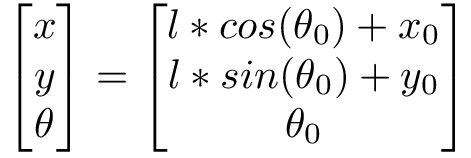An arc segment of lengthwith angular velocityis defined by: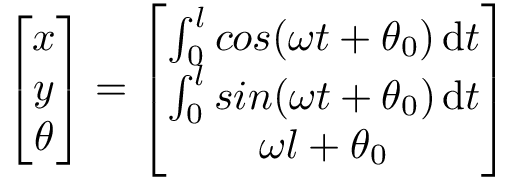More intersetingly, a spiral segment of lengthwith initial angular velocity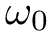and angular acceleration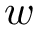is defined by: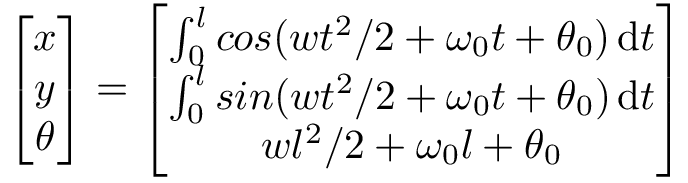The solution to these equations is left as an exercise to the reader. It is sufficient to note that while the equations for the linear and arc segments are closed-form and invertable, the equations for the spiral are neither.

By combining a spiral, an arc, and a second spiral the same length as the first, we can create a path that results in a net angular change, but starts and ends with zero angular velocity. By combining two of these with opposing curvatures, we can create a smooth path that has an offset in both X and Y, but zero angular offset, and zero angular velocity at the start and end points.

By using these two path constructs to create primitives, we can guarantee that the meeting points between primitives always have zero angular velocity. This alllows us to describe the search lattice with only 3 variables (x, y and yaw), while still guaranteeing path smoothness. This should reduce the search time significantly compared to a planner that considers angular velocity as part of the search space.

To generate smooth primitives, we paremeterize our path on the length and angular acceleration of the first spiral, and the length of the first arc. Given the symmetry of the paths we’re generating, this is sufficient to completely describe the path.

Since we can’t solve directly for the path parameters, we cheat and run a numeric optimization to find parameters that produce each primitive.

The current solution simply searches for all primitives whose end point is within one minimum turning radius of the robot’s start position. This produces a significant number of overlapping primitives: